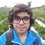# Pre-RMO 2014/20

What is the number of ordered pairs $(A, B)$ where $A$ and $B$ are subsets of $\{1, 2, \ldots, 5\}$ such that neither $A \subseteq B$ nor $B \subseteq A$ ?

This note is part of the set Pre-RMO 2014Note by Pranshu Gaba
6 years, 9 months ago

This discussion board is a place to discuss our Daily Challenges and the math and science related to those challenges. Explanations are more than just a solution — they should explain the steps and thinking strategies that you used to obtain the solution. Comments should further the discussion of math and science.

When posting on Brilliant:

• Use the emojis to react to an explanation, whether you're congratulating a job well done , or just really confused .
• Ask specific questions about the challenge or the steps in somebody's explanation. Well-posed questions can add a lot to the discussion, but posting "I don't understand!" doesn't help anyone.
• Try to contribute something new to the discussion, whether it is an extension, generalization or other idea related to the challenge.

MarkdownAppears as
*italics* or _italics_ italics
**bold** or __bold__ bold
- bulleted- list
• bulleted
• list
1. numbered2. list
1. numbered
2. list
Note: you must add a full line of space before and after lists for them to show up correctly
paragraph 1paragraph 2

paragraph 1

paragraph 2

[example link](https://brilliant.org)example link
> This is a quote
This is a quote
    # I indented these lines
# 4 spaces, and now they show
# up as a code block.

print "hello world"
# I indented these lines
# 4 spaces, and now they show
# up as a code block.

print "hello world"
MathAppears as
Remember to wrap math in $$ ... $$ or $ ... $ to ensure proper formatting.
2 \times 3 $2 \times 3$
2^{34} $2^{34}$
a_{i-1} $a_{i-1}$
\frac{2}{3} $\frac{2}{3}$
\sqrt{2} $\sqrt{2}$
\sum_{i=1}^3 $\sum_{i=1}^3$
\sin \theta $\sin \theta$
\boxed{123} $\boxed{123}$

Sort by:

is it 30???

- 6 years, 9 months ago

How many elements can A or B can have? Only 1 or more than one

If only 1 element ans $\to$ 20

Otherwise(counting every possibility) ans $\to$ 47

- 6 years, 9 months ago

Since $A$ and $B$ are subsets of $\{1, 2, 3, 4, 5\}$, they can have from 0 to 5 elements.

- 6 years, 9 months ago

Write a comment or ask a question... I think 52

- 6 years, 9 months ago

should be 1024 - 243 - 243 + 32 = 570

- 6 years, 9 months ago

ww margera. actually you permuted it...... {1,2} is Same as {2,1}

- 6 years, 9 months ago

Ordered pairs right? So the two should not be the same...

- 6 years, 9 months ago

@ww margera. actually you permuted it...... {1,2} is Same as {2,1}

- 6 years, 9 months ago

As @ww margera said, we can use principle of inclusion and exclusion.

$\text{Answer} = \text{total no. of ordered pairs} - (|A \subseteq B |+ |B \subseteq A|) + |A = B|$

where $|x|$ is no. of ordered pairs $(A, B)$ satifying condition $x$.

$\text{Answer}= 2^{10} - ( 3^5 + 3^5) + 2^5 = \boxed{570}$

- 6 years, 9 months ago

How 2^10, 3^5, 2^5, I didn't get that.

- 2 years, 11 months ago

why should it be 2^10......i think it should be ${2^5 \choose 2}*2$

- 6 years, 9 months ago

Using rule of product, $A$ has $2^5$ choices, and even $B$ has $2^5$ choices, so total no. of ordered pairs = $2^5 \times 2^5 = 2^{10}$

- 6 years, 9 months ago

6

- 6 years, 9 months ago

570

- 5 years, 9 months ago

@Pranshu Gaba : Are you studying in resonance? Which Centre?

- 5 years, 9 months ago